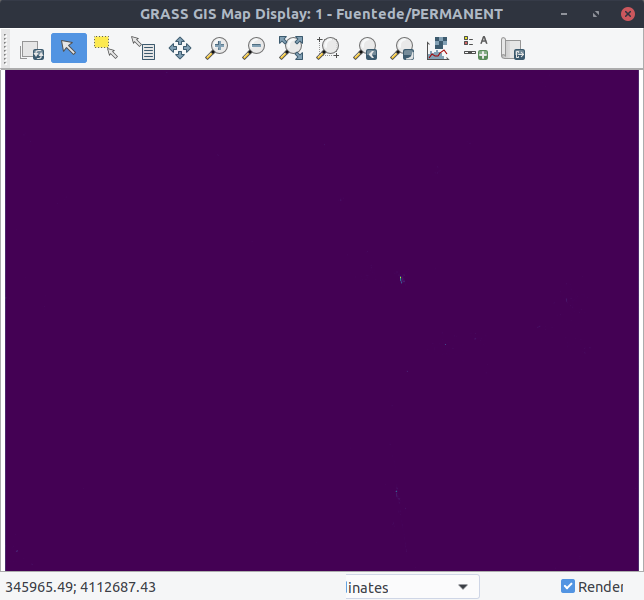# Spatio-temporal Calculation with Sentinel Data¶

In the previous step we create a spatio-temporal dataset in unit dB. Now, we will convert the dB units to linear unit with `t.raster.mapcalc`. To do this we launch the mapcalc GUI with:

```\$ t.rast.mapcalc
```

We choose the space time raster dataset that we created in the previous step Intensity. To convert the unit [dB] in [linear] we use the formula:

```pow(10, (dB/10))
```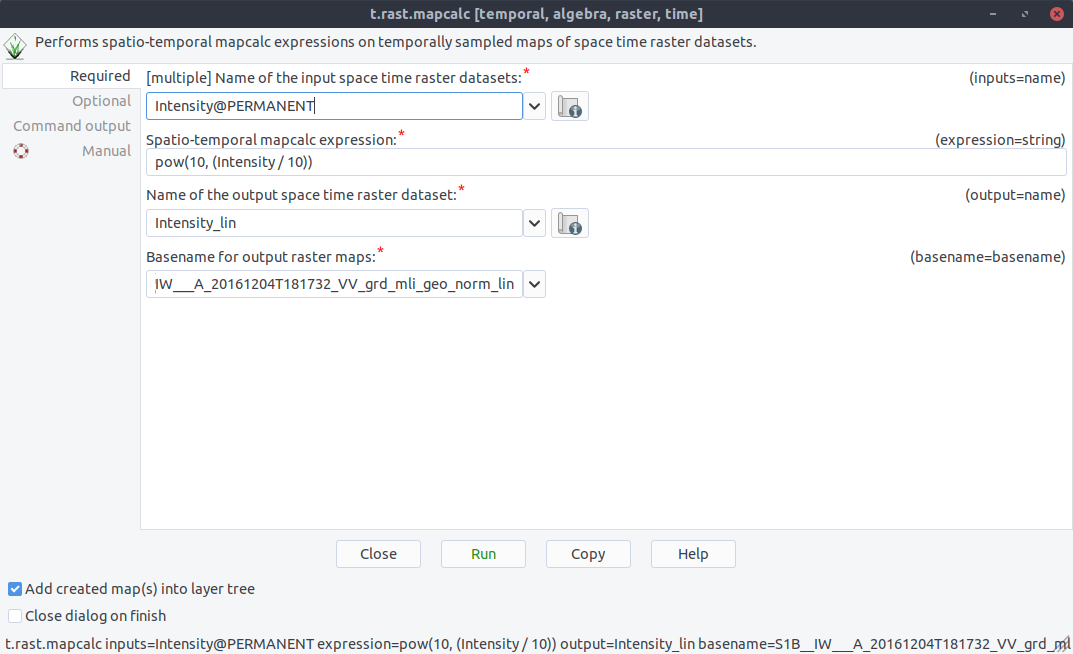Now, we will click `Run`: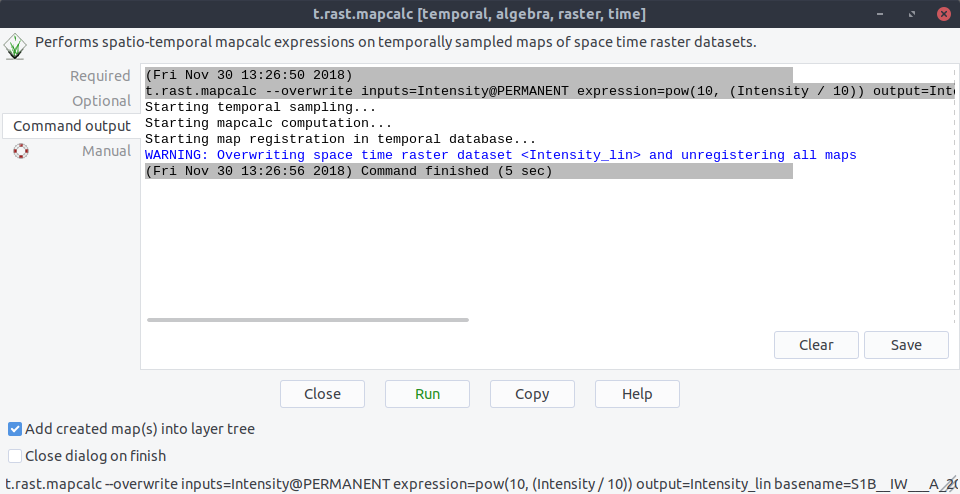If we now list all spatio-temporal dataset we see two entries: The one for `Intensity` and the another one `Intensity_lin` that we created in with `t.raster.mapcalc`: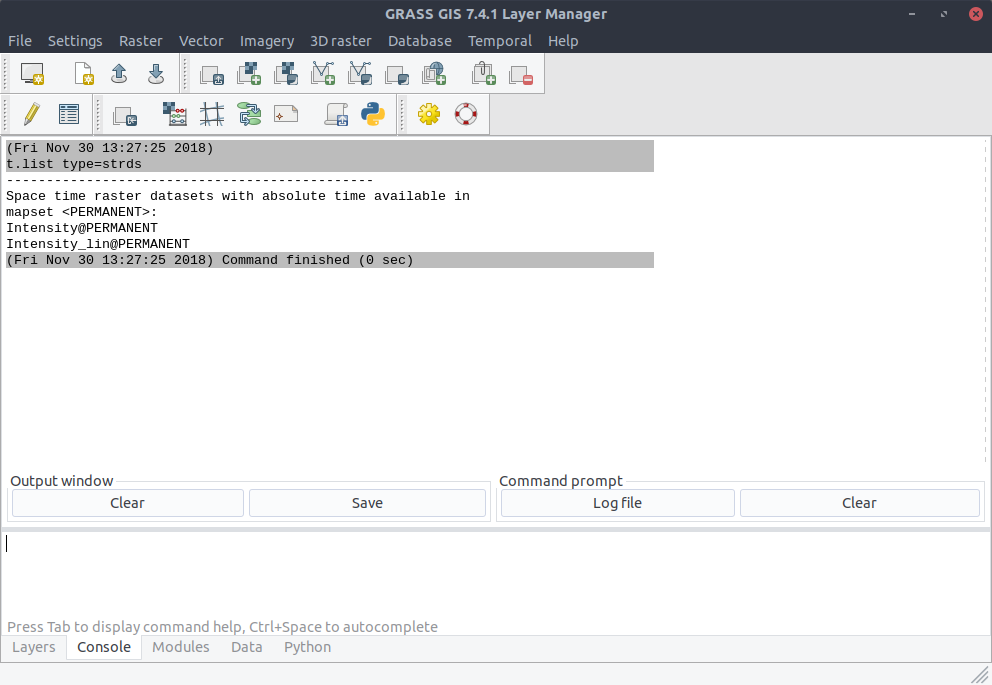We can also import the dataset into the display. Here is the dataset in unit [dB]: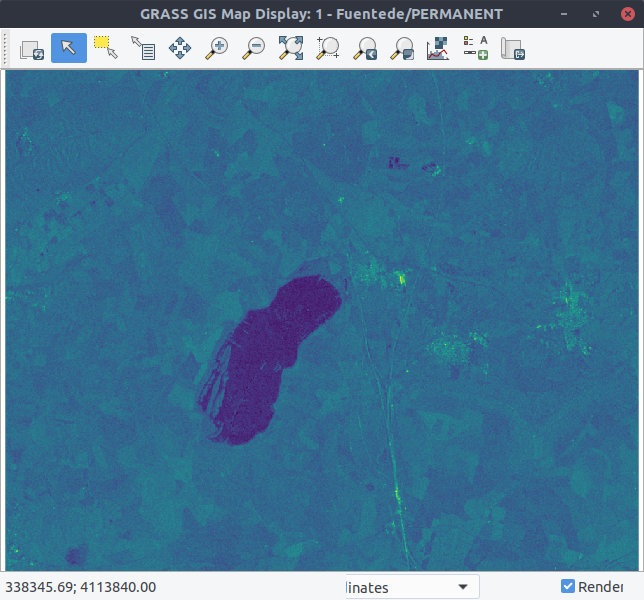And here is the dataset in unit [linear]: